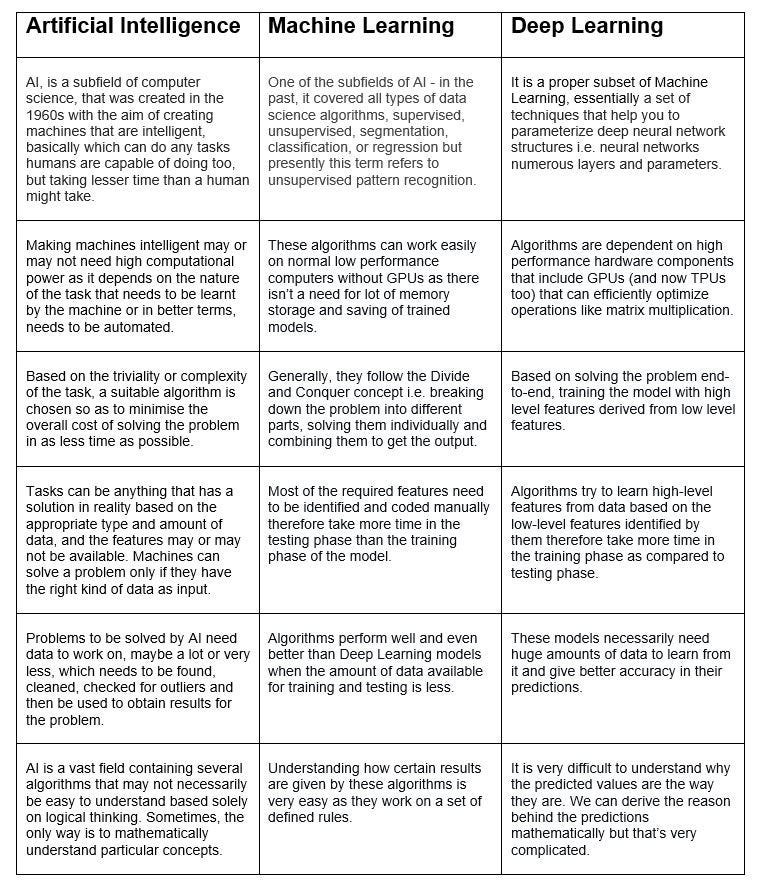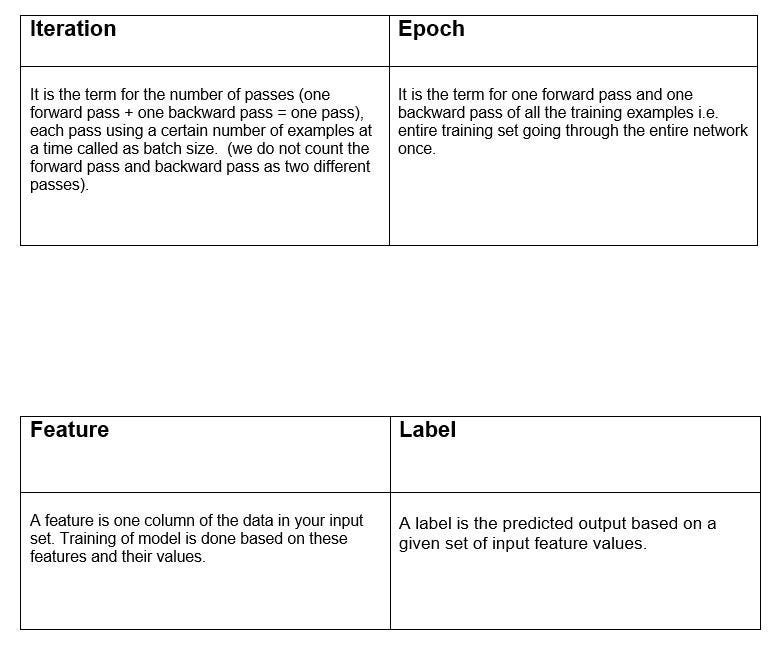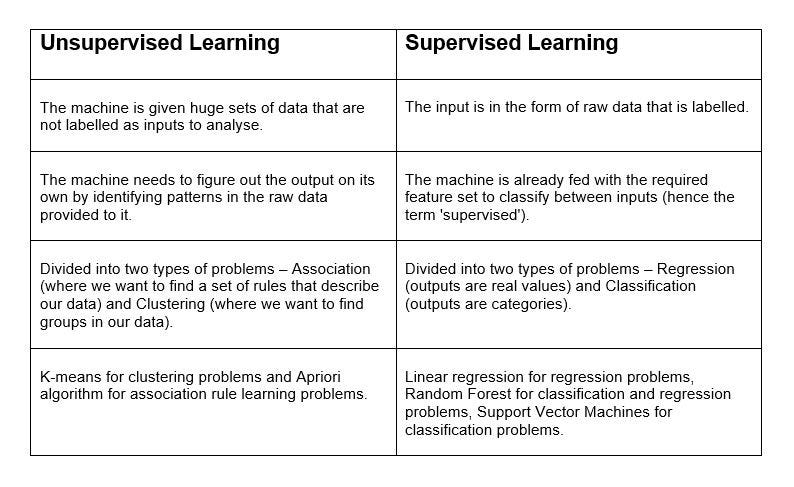Topics: AI | Data Science | Data Visualization | Deep Learning | Machine Learning | NLP | Python | R | Statistics

KDnuggets Home » News » 2017 » Oct » Tutorials, Overviews » Neural Networks: Innumerable Architectures, One Fundamental Idea ( 17:n39 )

# Neural Networks: Innumerable Architectures, One Fundamental Idea

http likes 79

At the end of this post, you’ll be able to implement a neural network to identify handwritten digits using the MNIST dataset and have a rough time idea about how to build your own neural networks.

Before getting into this post, I want to thank all you guys out there who liked and appreciated my first post! I received a lot of messages and comments appreciating my work. Thank you so much!

### Can I follow this post without having installed Tensorflow?

Yes! You can easily understand everything about this post and get into the installation to implement things yourself after understanding it because I’ve explained literally every single line and every bit of syntax in the code.

→ You may skip this part of the post and jump to the next question if you already have installed Tensorflow!

Remember to refer to this part to get everything you need to successfully install Tensorflow :-

Basically, we’ll be using Tensorflow and Command Prompt for understanding a neural network and its implementation​. Tensorflow installation used to be a hectic job but it’s got a bit easier now. Follow these links -

This video is self sufficient -

GPU version video tutorial -

If you haven’t had much experience with command prompt, here’s a good way to start -

### Why am I reading this post?

Some neural network models are not used anymore as researchers have developed better models with these forming the basis, but they’re still essential as the new developments have their roots in these concepts and we can’t understand new concepts without knowing the old ones.

I’ll try to keep the obsolete concepts short yet enough to get them into your heads permanently. Let’s get done with them and move on to the giants of deep learning! So, at the end of this post, you’ll be able to implement a neural network to identify handwritten digits using the MNIST dataset and have a rough time idea about how to build your own neural networks.

### Just start, will you?

Alright then! We’ll start off by understanding certain important terms before jumping into the code and visualizing the working of a basic neural network. Here we go!

Remember how we drew columns to differentiate between things in examination papers during school days? Let’s jot down differences between terms most people seem to be utterly confused about. The only twist this time would be to not include unnecessary points just to increase the total number of points for more marks!Difference between AI, ML and Deep LearningDifference between fundamental terms often used interchangeably!Difference between Supervised Learning and Unsupervised Learning

### Some Important Terms...

Artificial Neural Networks —

• Biologically inspired models to represent how we imagine the human brain functions.
• Form the roots of deep learning.
• The goal is to the most accurate prediction of an unknown function.
• Formed by interconnected neurons.
• These neurons have weights, and bias which are altered during the network training depending upon the cost function.

Nodes —

• Also called neurons and similar to the neurons in the human brain.
• Basic structural units of any neural network.
• A group of nodes together form a layer.
• A neuron receives an input from the previous layer’s neurons, processes it in its own layer and transfers the output to the next layer.

Weights —

• Any values that are multiplied by the input of previous layer’s node.
• Each connection has a unique weight associated to it.
• Higher the weight, more valuable is the input for an accurate prediction.

Biases —

• Linear components added to the product of weight and input from the previous layer neurons before passing it through the activation function in its own layer.
• A layer without a bias would mean just the multiplication of an input vector with a matrix of weights ( i.e. using a linear function) and thus an input of all zeros will always be mapped to an output of all zeros.
• They are different from bias nodes that are just a single mode for every layer which always has its value equal to one.

Hidden layers —

• Layers of nodes between the input later and the output layer where the processing of data actually takes place.
• A Deep NN has the number of hidden layers is greater than or equal to 2, else it is a regular one.
• We can keep adding layers until the test error does not improve anymore.

Tensors —

• Typed multidimensional array, basically an array having a bunch of values of any sizes.
• Tons of matrix manipulation libraries are predefined to work on them.
• In Tensorflow, we define a model in abstract terms by making a computation graph.
• The session runs the graph when it’s ready and everything is done on the graph at the backend.

• Gradient is the ratio of the rate of change of parameters to the error produced by it while learning and training itself to make predictions accurately.
• The process of minimizing the error is called gradient descent.
• Descending a gradient has two aspects: choosing the direction to go in (momentum) and choosing the size of the step (learning rate).

Activation function —

• Non linear functions that work on the sum of product of input and weight, and the bias and transfer the result to the next layer neurons.
• Used to make sure that the representation in the input space is mapped to a different space in the output.
• Usually continuous and derivative all over, so that it can capture notable behaviors among the linear inputs passed to it.
• Rectified Linear Unit (ReLU) and Hyperbolic Tangent are some famous activation functions.

Backpropagation —

• When the output for one iteration is computed, the error is calculated between the predicted value and expected value.
• This error is sent back into the network with the gradient of the cost function to alter the weights accordingly.
• These weights are then updated so that the errors in the following iterations are reduced.
• This updating of weights using the gradient of the cost function is called backpropagation.

Optimizers —

• Optimization is the process of finding the values of parameters for which the value of cost function turns out to be minimum and the algorithms that carry out this process are called optimizers.
• Most widely used ones are Adam, Adagrad and Stochastic Gradient Descent Optimizers among several others out there. (We’ll discuss about which one to use when and why in the upcoming posts!)

Softmax Function —

• It is defined as a generalization of the logistic function (S- shaped sigmoid function) that squashes a K-dimensional vector of arbitrary real values to a K-dimensional vector of real values in the range [0, 1] that add up to 1.

Any other terms required to understand the code and basic neural networks are in the Python code’s comments for your convenience.

Top Stories Past 30 Days
Most Popular
Most SharedGet KDnuggets, a leading newsletter on AI, Data Science, and Machine Learning